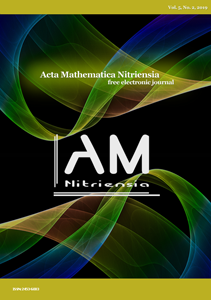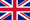## Papers & Abstracts## Acta Mathematica NitriensiaVol. 5, No. 2, 2019

### Content

 Viliam Ďuriš, Peter Korman: Root Approximation in Matlab Computational EnvironmentFull paper

Abstract: The task of solving non-linear equation occurs in practically all engineering disciplines. In the case of one equation, it is always possible to approximate the root within the required accuracy and to use some convergent method. The article deals with three basic convergent methods for roots approximation, namely bisection, tangent method and chord method, which are implemented and tested on several tasks in solving non-linear equations in Matlab computational environment as a suitable tool for implementation of various numerical methods.

Keywords: root approximation, bisection, Newton's method, false-proposition, Matlab

Classification: 49M15, 65H04

Pages: 1 - 10

DOI: 10.17846/AMN.2019.5.2.1-10

Full paper